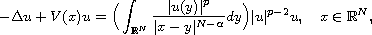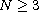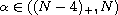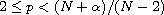Electron. J. Differential Equations, Vol. 2017 (2017), No. 52, pp. 1-14.

### Ground state solutions for Choquard type equations with a singular potential Tao Wang

Abstract:
This article concerns the Choquard type equationwhere,,and V(x) is a possibly singular potential and may be unbounded below. Applying a variant of the Lions' concentration-compactness principle, we prove the existence of ground state solution of the above equations.

Submitted June 6, 2016. Published February 21, 2017.
Math Subject Classifications: 35A15, 35A20, 35J20.
Key Words: Choquard equation; singular potential; ground state solution; Lions' concentration-compactness principle.

Show me the PDF file (273 KB), TEX file for this article.Tao Wang College of Mathematics and Computing Science Hunan University of Science and Technology Xiangtan, Hunan 411201, China email: wt_61003@163.com

Return to the EJDE web page